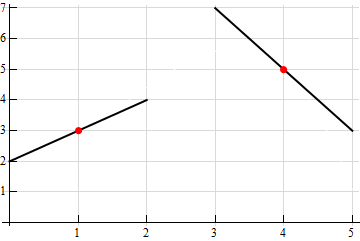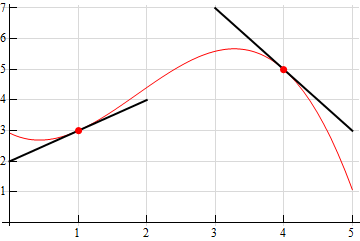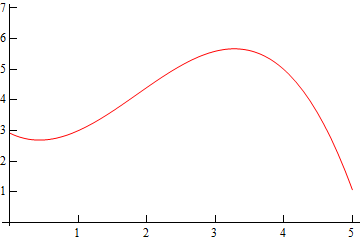Paul's Online Notes
Home / Calculus I / Derivatives / Interpretation of the Derivative
Show Mobile Notice Show All Notes Hide All Notes
Mobile Notice
You appear to be on a device with a "narrow" screen width (i.e. you are probably on a mobile phone). Due to the nature of the mathematics on this site it is best views in landscape mode. If your device is not in landscape mode many of the equations will run off the side of your device (should be able to scroll to see them) and some of the menu items will be cut off due to the narrow screen width.

### Section 3.2 : Interpretation of the Derivative

3. Sketch the graph of a function that satisfies$$f\left( 1 \right) = 3$$, $$f'\left( 1 \right) = 1$$, $$f\left( 4 \right) = 5$$, $$f'\left( 4 \right) = - 2$$.

Show All Steps Hide All Steps

Hint : Remember that one of the interpretations of the derivative is the slope of the tangent line to the function.
Start Solution

First, recall that one of the interpretations of the derivative is that it is the slope of the tangent line to the function at a particular point. So, let’s start off with a graph that has the given points on it and a sketch of a tangent line at the points whose slope is the value of the derivative at the points.Show Step 2

Now, all that we need to do is sketch in a graph that goes through the indicated points and at the same time it must be parallel to the tangents that we sketched. There are many possible sketches that we can make here and so don’t worry if your sketch is not the same as the one here. This is just one possible sketch that meets the given conditions.While, it’s not really needed here is a sketch of the function without all the extra bits that we put in to help with the sketch.